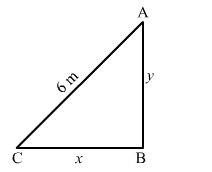# The top of a ladder 6 metres long is resting against a vertical wall on a level pavement,

Question:

The top of a ladder 6 metres long is resting against a vertical wall on a level pavement, when the ladder begins to slide outwards. At the moment when the foot of the ladder is 4 metres from the wall, it is sliding away from the wall at the rate of 0.5 m/sec. How fast is the top-sliding downwards at this instance?
How far is the foot from the wall when it and the top are moving at the same rate?

Solution:

Let the bottom of the ladder be at a distance of x m from the wall and its top be at a height of y m from the ground.Here,

$x^{2}+y^{2}=36$

$\Rightarrow 2 x \frac{d x}{d t}=-2 y \frac{d y}{d t}$            .....(1)

When $x=4, y=\sqrt{36-16}=2 \sqrt{5}$

$\Rightarrow 2 \times 4 \times 0.5=-2 \times 2 \sqrt{5} \frac{d y}{d t}$       $\left[\because \frac{d x}{d t}=0.5 \mathrm{~m} / \mathrm{sec}\right]$

$\Rightarrow \frac{d y}{d t}=\frac{-1}{\sqrt{5}} \mathrm{~m} / \mathrm{sec}$

From eq. $(1)$, we get

$2 x \frac{d x}{d t}=-2 y \frac{d y}{d t}$      $\left[\because \frac{d x}{d t}=\frac{d y}{d t}\right]$

$\Rightarrow x=-y$

Substituting $x=-y$ in $x^{2}+y^{2}=36$, we get

$x^{2}+x^{2}=36$

$\Rightarrow x^{2}=18$

$\Rightarrow x=3 \sqrt{2} \mathrm{~m}$